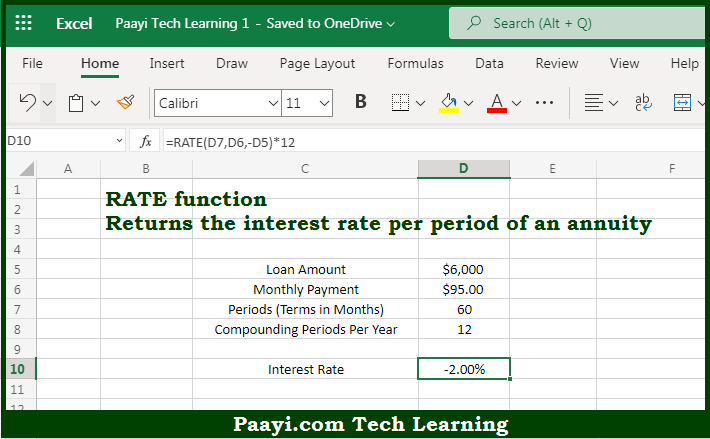# Learn How to Use Microsoft Excel RATE Function

Written by | 0 Comments | 352 Views

In this article, you will learn how to use the Microsoft Excel RATE function and its prime function in Microsoft Excel. You will also get to know the Microsoft Excel RATE function return value and syntax with the help of some examples.

Microsoft Excel RATE Function

The main purpose of the Microsoft Excel RATE function is to get the interest rate per period of the annuity. That implies, with the help of the RATE function you can able to return the interest rate per period of an annuity. The RATE can be used to calculate the periodic interest rate, then multiply as required to derive the annual interest rate. It should be noted that the RATE function calculates by iteration. So, with the help of the RATE function, you can able to get the interest rate per period of the annuity.

Return Value of RATE Function

The return value will be the interest rate per period.

Syntax of RATE Function

=RATE(nper, pmt, pv, [fv], [type], [guess])

Where the arguments:

• nper: This is the total number of payment periods.
• pmt: This is the payment made each period.
• pv: This is the present value or the total value of all the loan payments presently.
• fv: This is the cash balance you want to get after the last payment is made. In case not, it is assumed to be zero (optional).
• type: This is used when payments are due, where 0 = end of the period, and 1 = beginning of the period. The default is 0 (optional).
• guess: This is your guess on rate, default is 10% (optional).

How to Use Microsoft Excel RATE Function?So we know that Microsoft Excel RATE function you can able to get the interest rate per period of the annuity. That implies, with the help of the RATE function you can able to return the interest rate per period of an annuity. The RATE can be used to calculate the periodic interest rate, then multiply as required to derive the annual interest rate. It should be noted that the RATE function calculates by iteration. So, with the help of the RATE function, you can able to get the interest rate per period of the annuity.